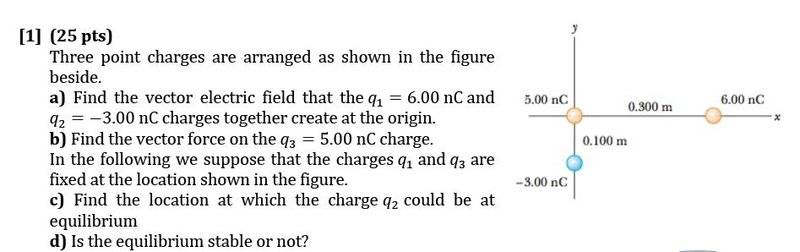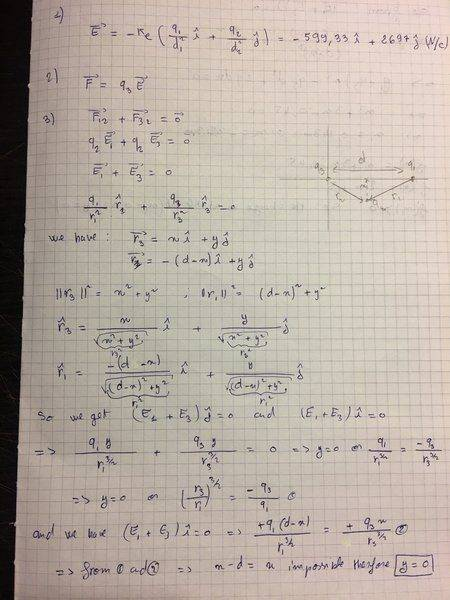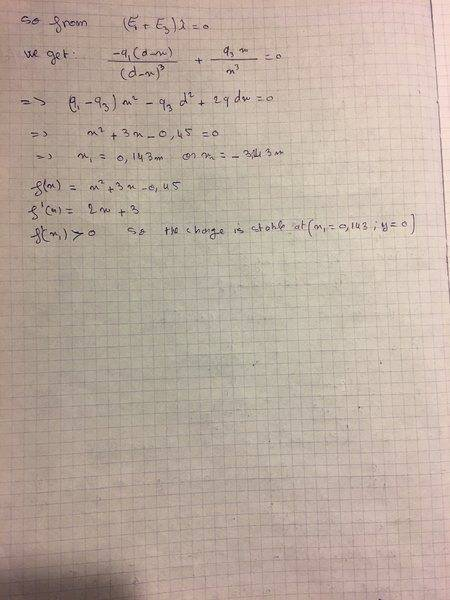# Equilibrium stability

• Mary1995

## Homework Statement

The equation x^2+3x-0.45=0 has two solutions x1=0.14 and x2=-3.14, these are supposed to be the locations at which the electric charge is in equilibrium with two other charges, should I use the first derivative to see at which location the charge will be stable?

x^2+3x-0.45=0

## The Attempt at a Solution

f’=2x+3, f’(x1)>0 so the charge is stable, is my reasoning correct?

## Homework Statement

The equation x^2+3x-0.45=0 has two solutions x1=0.14 and x2=-3.14, these are supposed to be the locations at which the electric charge is in equilibrium with two other charges, should I use the first derivative to see at which location the charge will be stable?

x^2+3x-0.45=0

## The Attempt at a Solution

f’=2x+3, f’(x1)>0 so the charge is stable, is my reasoning correct?
Welcome to the PF, Mary.Could you post the full problem statement, along with a diagram of the charge placements? And what you have derived is the net Coulomb force on a test charge due to two other fixed charges?

Hi Berkaman, thanks for your response, here is the full exercice:Thank you!

#### Attachments

Hi Berkaman, thanks for your response, here is the full exercice:and here is my answer: View attachment 214849 View attachment 214850 View attachment 214851

Thank you!
I'm having trouble following your handwritten solution. Could you summarize the answers you got? And are you saying that the charge is in equilibrium and stable in a position between the two other charges and with y=0, or am I misreading that part? If that is what you are saying, I don't think it is correct...

I first didn't know where the charge would be located at equilibrium so I tried to get a general formulas from E1j+E3j=0 then E1i+E3i=0
from the first equation I deduced that y would be zero, so the charge would be located at the x-axis then from the second equation I got the locations on the x axis, two results were found, I have to choose one at which the charge would be stable so I thought about the first derivative.

I first didn't know where the charge would be located at equilibrium so I tried to get a general formulas from E1j+E3j=0 then E1i+E3i=0
from the first equation I deduced that y would be zero, so the charge would be located at the x-axis then from the second equation I got the locations on the x axis, two results were found, I have to choose one at which the charge would be stable so I thought about the first derivative.
I don't think that's right so far. What vector answers did you get for parts a) and b) ?

And for c), think about it intuitively first. The test charge is positive, and the two other charges are positive and negative. The test charge will be attracted to one and repelled by the other. Those forces have to cancel out in order for the net force to be zero. If the test charge is not on the line going through those two particles, how can the net vector forces be zero...?

isn't the test charge q2=-3.0 nC <0?? it is supposed to be between the two positive charges on the line x, I seems logic!

isn't the test charge q2=-3.0 nC <0?? it is supposed to be between the two positive charges on the line x, I seems logic!
Oh, my bad, sorry. For parts a) and b), they were asking about the field and force at the origin where q3 is. Then you are right, for part c) they switched and asked about movning q2. Sorry that I caused confusion.

And yes, in that case, q2 needs to be somewhere on the x-axis, between the two + charges. Sorry. So did you still get two solutions for the possible x-position of q2? Can you graph approximately what the force field looks like around the point(s) where the net force is zero?

Hmm, well that shows a zero between the origin and x=0.3m which is where the 2 positive charges are located. But the rest of the plot doesn't make sense to me. What did you get for the euation of the net force on the negative q3 charge as a function of position x? It seems like it would go to infinity at the x position of each of the two positive charges, no?